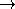advanced## RandomConvexSetTraits_2

### Definition

The concept RandomConvexSetTraits_2describes the requirements of the traits class for the function random_convex_set_2.

### Types

 RandomConvexSetTraits_2::Point_2 point class. RandomConvexSetTraits_2::FT class used for doing computations on point and vector coordinates (has to fulfill field type requirements). RandomConvexSetTraits_2::Sum AdaptableBinaryFunction class: Point_2  × Point_2Point_2. It returns the point that results from adding the vectors corresponding to both arguments. RandomConvexSetTraits_2::Scale AdaptableBinaryFunction class: Point_2  × FTPoint_2. Scale(p,k) returns the point that results from scaling the vector corresponding to p by a factor of k. RandomConvexSetTraits_2::Max_coordinate AdaptableUnaryFunction class: Point_2FT. Max_coordinate(p) returns the coordinate of p with largest absolute value. RandomConvexSetTraits_2::Angle_less AdaptableBinaryFunction class: Point_2  × Point_2bool. It returns true, iff the angle of the direction corresponding to the first argument with respect to the positive x-axis is less than the angle of the direction corresponding to the second argument.

### Operations

 Point_2 t.origin () const return origin (neutral element for the Sum operation).advanced